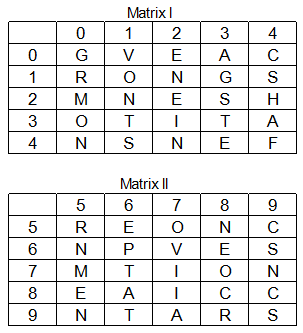## SSC MTS Reasoning Test 7

Instructions

For the following questions answer them individually

Q 1

If a mirror is placed on the line AB, then which of the answer figure is the right image of the given figure ?

Q 2

For the following questions
Find the odd word/letters/number pair from the given alternatives

Q 3

In the following question, four number pairs are given. The number on left side of (-) is related to the number of the right side of (-) with some
Logic/Rule/Relation. Three are similar on basis of same Logic/Rule/Relation. Select the odd one out from the given alternatives.

Q 4

Identify the set for the word 'STAR'.Q 5

Ina certain code, RAINIER is written as 9195959. How will POEM be written in that code?